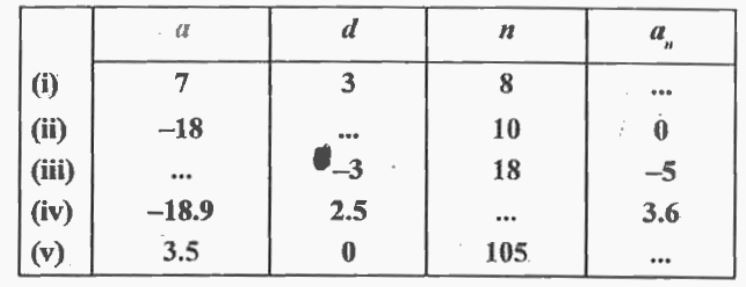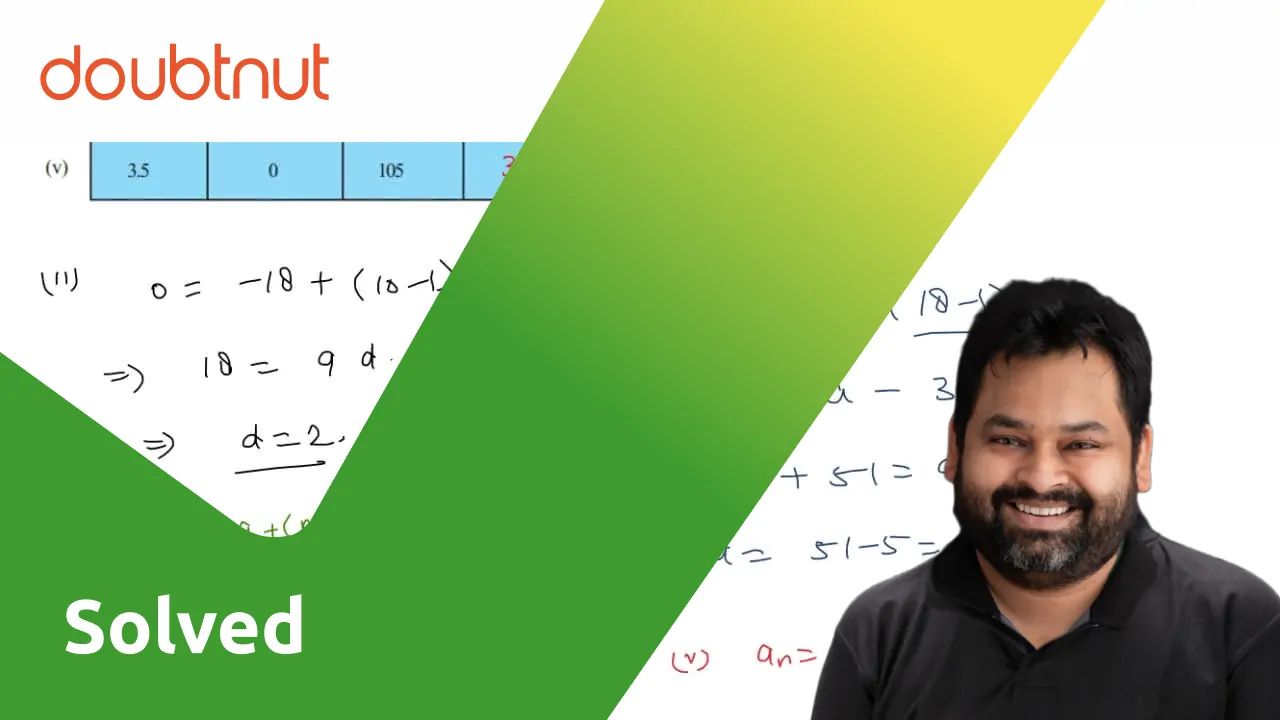Doubtnut App - Get 50 Lakh+ video solutions better than GoogleInstall Now

# Fill in the blanks in the following table, given that a is the first term, d the common difference and anthe nth term of the AP:Text Solution
Verified by Experts

##<br>TheforμlaforthenthtermofanAPis,a_n = a + (n - 1)d.<br>i)Given:a = 7, d = 3, n = 8<bra_n= a + (n - 1)d<br>a_8 = 7 + (8 - 1)3<br>= 7 + 7 times 3<br>= 7 + 21<br>= 28<br>a_n = 28<br>ii)Given:a = -18, aₙ = 0, n = 10<br>a_n= a + (n - 1)d<br>0 = - 18 + (10 - 1)d<br>0 = - 18 + 9d<br>9d = 18<br>d = 2<br>iii)Given:d = -3, a_n = - 5, n = 18<br>- 5 = a + (18 - 1)(- 3)<br>- 5 = a + 17 × (- 3)<br>a = 46<br>iv)Given:a_n = 3.6, d = 2.5, a = - 18.9<br>a_n = a + (n - 1)d<br>3.6 = - 18.9 + (n - 1) 2.5<br>3.6 + 18.9 = 2.5 (n - 1)<br>22.5 = 2.5 (n - 1)<br>n - 1 = 22.5 / 2.5<br>n - 1 = 9=>n = 10<br>v)Given:a = 3.5, d = 0, n = 105<br>a_n = a + (n - 1)d<br>a_n = 3.5 + (105 - 1) (0)<br>a_n = 3.5 + 104 × 0<br>a_n = 3.5